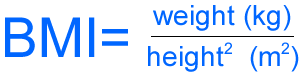## CS 16, Spring 2012 Programming Project 1

### Due: Monday, July 23, 11:59pm Worth: 4% of final grade

 Before you begin: If you have the textbook, read through the end of chapter 2. Pay special attention to the C programs (particularly pages 21, 63 and 74). Read all of the instructions below to make sure you understand the problems completely before attempting to solve them. For each problem, plan to accomplish all parts of the 5-step problem-solving process outlined in the text: Problem statement - okay, so this part is done already, but you still have to verify that you understand the problems. Input/output description - this part is done too: see the sample runs below. Also you can execute (but not read) our solutions at CSIL to learn more about how we expect your solutions to work. Run either solution as follows, substituting either `bmi` or `logbase` for `PGMNAME`: `-bash-4.2\$ ~kyledewey/cs16/p1/PGMNAME` Just one clarification: print all floating point values formatted with 1-digit precision. Hand example - do this part to know exactly what calculations are necessary, and the order in which those calculations must be done. Algorithm development - we give you some clues about the overall program structures below, but you must apply what you learned from the hand example to get the calculations right. In the text's process, this step also includes getting the programs typed in and working! Testing - Don't skip this step. Find and fix your errors before turning in.
1. Write a C program to calculate a person's body mass index (BMI) for a user.At left is the formula for calculating BMI, given a person's weight in kilograms and height in meters. Your program will let the user enter weight in pounds and height in inches, and then convert to the metric units to calculate BMI.
• The program must behave and produce results exactly like our solution's behavior and results. Here are two sample runs (user input is boldface):
```bash-4.2\$ ./bmi
enter pounds and inches: 175 69
BMI is 25.8
-bash-4.2\$ ./bmi
enter pounds and inches: 118.5
64.2
BMI is 20.2
bash-4.2\$```
If you wonder what the results mean, see this BMI chart (thanks to Wikipedia).
• The source code file must be named `bmi.c` and we suggest you create a directory named `~/cs16/p1` to store it in.
• Type a valid C comment at the beginning of the file that includes your name and the date the program is written. (This instruction is IMPORTANT, and it applies to all of your future programs too!)
• Remember to include the standard input-output library.
• Part of the problem converts pounds to kilograms and inches to meters. We used the following symbolic constants (you may copy them and paste into your solution if you want):
```/* pounds per kilogram and inches per meter */
#define  PPKG   2.20462262
#define  IPM    39.3700787```
• Properly define (and conclude) a `main` function that carries out your algorithm's steps.
• Compile the program using `make` (just like you do with programs in lab), and test it with various input data to make sure it works correctly.
2. Write another C program to calculate a logarithm for a user, where the user enters both the original value and the base for which the logarithm is expressed. If you have the textbook, see Problems 41 and 42 on page 82 of the Etter text, and especially the formula shown above those problems. Your solution will actually be a general version that solves both of problems 41 and 42, plus infinite more like them. If you don't have the textbook, you may wish to consult http://www.sosmath.com/algebra/logs/log4/log43/log43.html and http://www.cplusplus.com/reference/clibrary/cmath/log/.
• The program must behave and produce results exactly like our solution's behavior and results. Here are two sample runs (user input is boldface):
```-bash-4.2\$ ./logbase
enter value and desired base: 1024 2
log of 1024.0 to base 2 is 10.0
-bash-4.2\$ ./logbase
enter value and desired base: 177.4 10
log of 177.4 to base 10 is 2.2
bash-4.2\$```
• The source code file must be named `logbase.c` and we suggest you store it in `~/cs16/p1` with your part 1 solution.
• If you have the textbook, see Section 2.7 in the textbook, and include all necessary standard libraries. (If you don't, consult the links.)
• At CSIL, it is likely that `make` will not properly complile your program. Probably you will need to use `gcc` with the `-lm` option as follows:
`-bash-4.2\$ gcc -o logbase -Wall logbase.c -lm`
3. Go to CSIL (in person unless you can manage this step remotely without any assistance from us). Open a terminal window, `cd` to the same directory as your source code files, then type the following:
`turnin p1@cs16 bmi.c logbase.c`

### Optional Challenges

Do not change bmi.c or logbase.c to accomplish these challenges. Write new programs instead. Do not turn in these challenges - they are for practice and to learn.

• Write a version of bmi.c that lets the user enter height as feet plus inches, as in 5'10" and then calculates total inches (or meters) from that information before calculating bmi.
• Write a version of bmi.c focused on users that prefer kilograms and meter units. Let the user enter the data in those units instead.
• Along with the regular BMI results, print some guidance that will help the user understand the results. For instance, print a table with the following data:
• Underweight: <18.5
• Normal weight: 18.5-24.9
• Overweight: 25-29.9
• Obesity: BMI of 30 or greater
• Use the data from the previous challenge, and employ an appropriate selection structure to tell the user the BMI class that results. For example, if the BMI result is 26.7, then the program should print a message that indicates the subject is overweight.
• If you have the textbook, try any of the other programming problems at the end of Etter chapter 2, pp. 81-83.